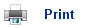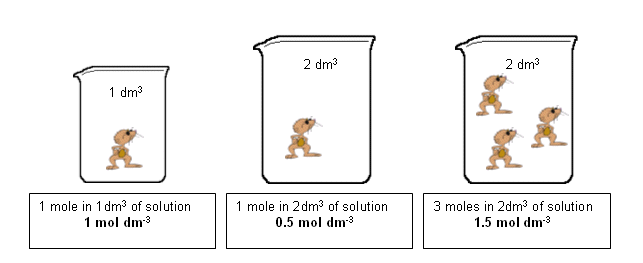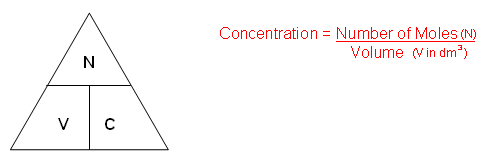# Concentrations of solutions

• In chemistry the mole is the main unit of the amount of substance that is used.
• The number of moles of solute present in a volume needs to be known by chemists (the concentration of the solution).
• The concentration of a solution is measured in mols per cubic decimetre (mol dm-3).
• One mole of any solute in a 1 litre volume has a concentration of 1 mol dm-3, for example 6.02 x 1023 particles of NaOH in a one litre solution, will have a concentration of 1 mol dm-3.• The concentration of a volume is worked out by dividing the number of moles by the volume:Example:

We have 250 cm3 of a solution of sodium hydroxide whose concentration is 2 mole dm-3. How many moles of sodium hydroxide do we have?

Rearrange the formula: Number of Moles (N)= Concentration (C) x Volume (V in dm3)
Change the units:
250 cm3 is the same as 0.25 dm3 (by dividing by 1000).
Substitute the values into the formula:
Number of Moles = 2 x 0.25
=0.5 moles

## Useful books for revision:

Revise AS Chemistry for Salters (Written by experienced examiners and teachers of Salter's chemistry)Revise AS Chemistry for Salters (OCR) (Salters Advanced Chemistry)Home